# 掌握14个Excel统计函数，让你的工作效率飞起来

## Excel初学者必会十大技巧 https://tech.wmzhe.com/article/50407.html

===============================以下是正文开始===============================

1、Count 函数

=COUNT(A\$1:A1)+1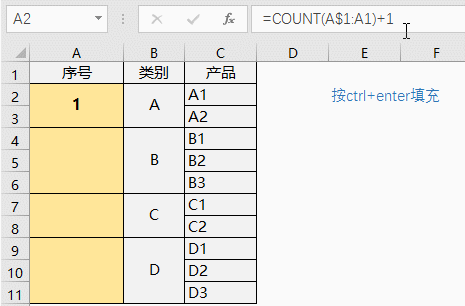2、Counta函数

=IF(COUNTA(D2:F2)>1,"达标","不达标")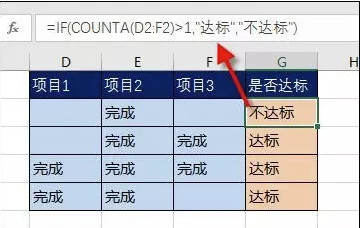3、Countif函数

=COUNTIF(Sheet15!A:A,A2)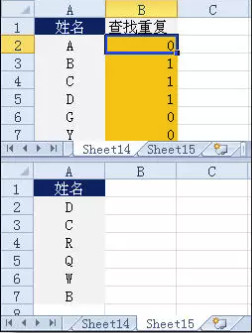4、Countifs函数

=COUNTIFS(B2:B8,"财务",C2:C8,"大专")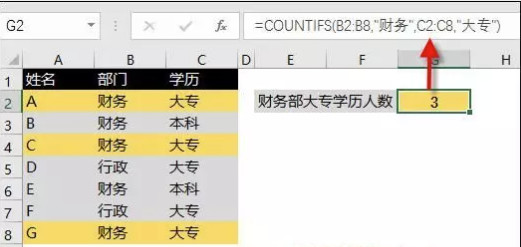5、Frequency函数

=FREQUENCY(D2:D8,{40,29})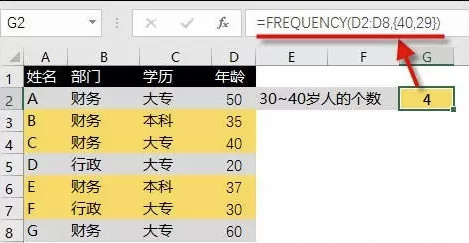6、Sumproduct函数

=SUMPRODUCT((--LEFT(YEAR(D2:D8),3)=199)*1)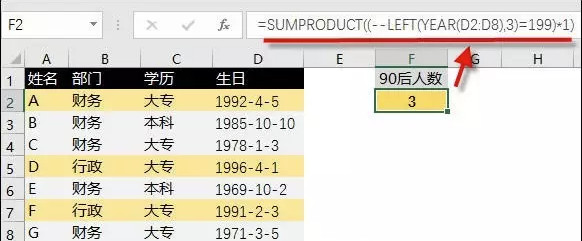7、Averageif函数

=AVERAGEIF(C2:C4,">0")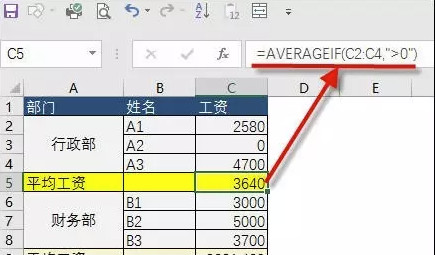8、Averageifs函数

=AVERAGEIFS(D:D,B:B,"财务",C:C,"大专")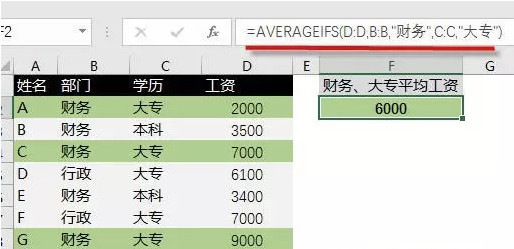9、Max函数、Maxifs函数

｛=MAX((B2:B8="财务")*D2:D8)｝

=MAXIFS(D2:D8,B2:B8,"财务")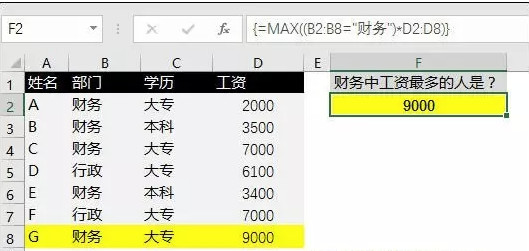10、Min函数、Minifs函数

=MINIFS(D2:D8,B2:B8,"财务")

｛=INDEX(A:A,MATCH(MIN(IF(B2:B8="财务",D2:D8)),D:D,0))｝11、Large函数

=LARGE(D:D,F2)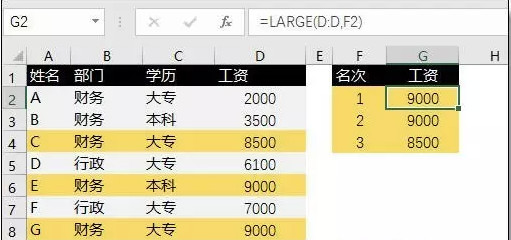12、Small函数

{=INDEX(C:C,SMALL(B\$2:B\$10=F\$1,ROW(\$2:\$10)),ROW(A1)))}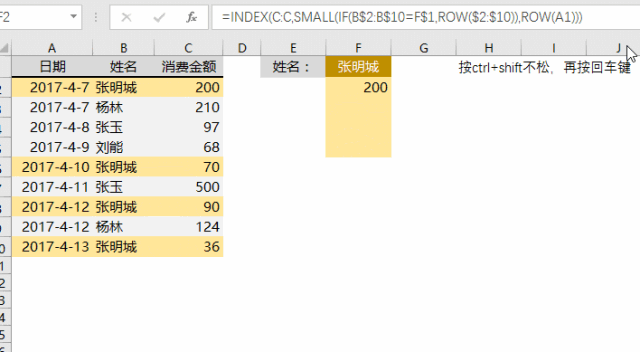13、Rank函数

=RANK(B2,B:B)14、Mode函数

=MODE(A2:A17)